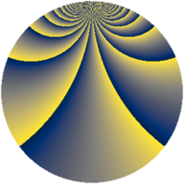# Properties

 Label 503.2.cLevel $503$ Weight $2$ Character orbit 503.c Rep. character $\chi_{503}(2,\cdot)$ Character field $\Q(\zeta_{251})$ Dimension $10250$ Newform subspaces $1$ Sturm bound $84$ Trace bound $0$

# Related objects

## Defining parameters

 Level: $$N$$ $$=$$ $$503$$ Weight: $$k$$ $$=$$ $$2$$ Character orbit: $$[\chi]$$ $$=$$ 503.c (of order $$251$$ and degree $$250$$) Character conductor: $$\operatorname{cond}(\chi)$$ $$=$$ $$503$$ Character field: $$\Q(\zeta_{251})$$ Newform subspaces: $$1$$ Sturm bound: $$84$$ Trace bound: $$0$$

## Dimensions

The following table gives the dimensions of various subspaces of $$M_{2}(503, [\chi])$$.

Total New Old
Modular forms 10750 10750 0
Cusp forms 10250 10250 0
Eisenstein series 500 500 0

## Trace form

 $$10250q - 249q^{2} - 249q^{3} - 285q^{4} - 245q^{5} - 237q^{6} - 247q^{7} - 239q^{8} - 280q^{9} + O(q^{10})$$ $$10250q - 249q^{2} - 249q^{3} - 285q^{4} - 245q^{5} - 237q^{6} - 247q^{7} - 239q^{8} - 280q^{9} - 233q^{10} - 239q^{11} - 239q^{12} - 245q^{13} - 223q^{14} - 231q^{15} - 263q^{16} - 243q^{17} - 217q^{18} - 229q^{19} - 195q^{20} - 215q^{21} - 233q^{22} - 241q^{23} - 181q^{24} - 280q^{25} - 215q^{26} - 237q^{27} - 185q^{28} - 217q^{29} - 159q^{30} - 219q^{31} - 185q^{32} - 209q^{33} - 167q^{34} - 187q^{35} - 203q^{36} - 237q^{37} - 161q^{38} - 197q^{39} - 135q^{40} - 209q^{41} - 117q^{42} - 221q^{43} - 147q^{44} - 167q^{45} - 167q^{46} - 195q^{47} - 141q^{48} - 234q^{49} - 145q^{50} - 177q^{51} - 179q^{52} - 211q^{53} - 83q^{54} - 165q^{55} - 125q^{56} - 177q^{57} - 205q^{58} - 189q^{59} - 27q^{60} - 193q^{61} - 143q^{62} - 159q^{63} - 185q^{64} - 155q^{65} - 97q^{66} - 201q^{67} - 155q^{68} - 113q^{69} - 123q^{70} - 153q^{71} - 21q^{72} - 213q^{73} - 83q^{74} - 161q^{75} - 97q^{76} - 139q^{77} - 43q^{78} - 191q^{79} + 3q^{80} - 188q^{81} - 97q^{82} - 161q^{83} + 67q^{84} - 159q^{85} - 121q^{86} - 121q^{87} - 143q^{88} - 155q^{89} + 115q^{90} - 137q^{91} - 119q^{92} - 123q^{93} - 99q^{94} - 181q^{95} + 67q^{96} - 229q^{97} - 63q^{98} - 85q^{99} + O(q^{100})$$

## Decomposition of $$S_{2}^{\mathrm{new}}(503, [\chi])$$ into newform subspaces

Label Dim. $$A$$ Field CM Traces $q$-expansion
$$a_2$$ $$a_3$$ $$a_5$$ $$a_7$$
503.2.c.a $$10250$$ $$4.016$$ None $$-249$$ $$-249$$ $$-245$$ $$-247$$